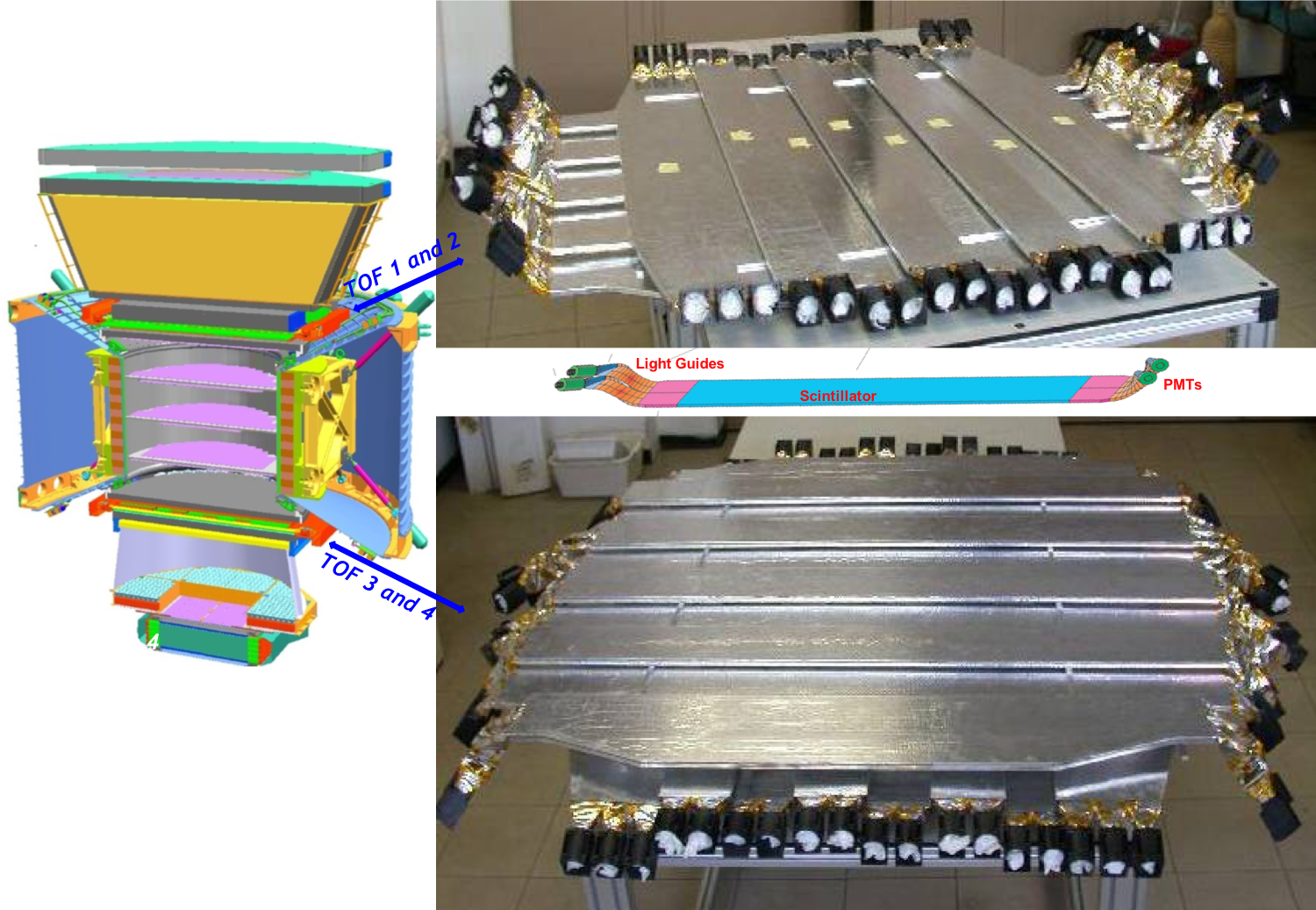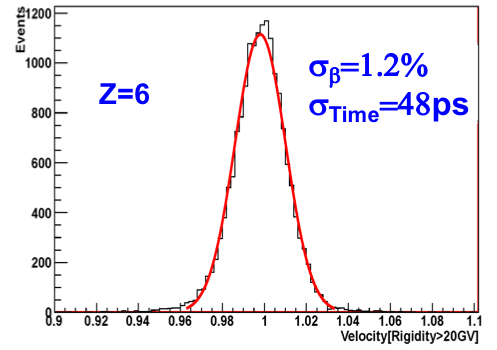# The Time of Flight (TOF) Counters

Two planes of Time of Flight (TOF) counters, shown in Figure 1, are located above and two planes below the magnet. Each plane contains eight or ten scintillating paddles. Each paddle is equipped with two or three photomultiplier tubes on each end for efficient detection of traversing particles. The coincidence of signals from all four planes provides a charged particle trigger. The TOF measures charge of particles and nuclei by mulitple measurements of the ionization energy loss. As seen from figure 2, the TOF clearly identify cosmic rays from proton ($Z=1$) to Zinc ($Z=30$) and beyond. The charge resolution is $\Delta{Z}{\simeq}0.05$ at $Z=1$. The average time resolution of each counter has been measured to be 160 ps, and the overall velocity ($\beta=v/c$) resolution of the system has been measured to be 4% for $\beta {\simeq}1$ and $Z=1$ particles, which also discriminates between upward- and downward-going particles. As seen in Figure 3, the timing resolution improves with increasing magnitude of the charge to a limit of $\Delta{t}$ ~ 50 ps and $\Delta{\beta}/\beta$ ~ 1% for $Z>5$ particles.Figure 1. The Time of Flight system.Figure 2. The charge distribution from proton (Z=1) to Zinc (Z=30) measured by the TOF.Figure 3. The velocity distribution of high rigidity ($R>20$ GV) carbon ($Z=6$) measured by the TOF is 1.2%, corresponding to a time resolution of 48 ps.